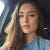# How to define table size in MS SQL database

To determine the size of tables in a database hosted on Microsoft SQL Server, you need to perform the following steps:
1. Connect to a database server using SQL Server Management Studio (SSMS)
2. Select a database whose table size you want to define
3. Execute SQL query:

`USE {database_name};`
`GO`

`SELECT`
`t.Name AS TableName,`
`s.Name AS SchemaName,`
`p.Rows AS RowCounts,`
`SUM(a.total_pages) * 8 AS TotalSpaceKB,`
`SUM(a.used_pages) * 8 AS UsedSpaceKB,`
`(SUM(a.total_pages) - SUM(a.used_pages)) * 8 AS UnusedSpaceKB`
`FROM`
`sys.tables t`
`INNER JOIN sys.indexes i ON t.object_id = i.object_id`
`INNER JOIN sys.partitions p ON i.object_id = p.object_id AND i.index_id = p.index_id`
`INNER JOIN sys.allocation_units a ON p.partition_id = a.container_id`
`LEFT OUTER JOIN sys.schemas s ON t.schema_id = s.schema_id`
`WHERE`
`t.Name NOT LIKE 'dt%'`
`AND t.is_ms_shipped = 0`
`AND i.object_id > 255`
`GROUP BY`
`t.Name, s.Name, p.Rows`
`ORDER BY`
`t.Name;`
`GO`

where:

• database_name — the name of the database, for which it is necessary to get the list of tables with sizes.

The size of the database tables will be specified by Kilobytes.

If you need to get a limited list of tables, for example, containing certain words in the name, you can shorten the output by adding a condition (t.Name Like ‘%Filter%’) to the WHERE construct

`WHERE`
`t.Name NOT LIKE 'dt%'`
`AND t.Name Like '%Filter%'`
`AND t.is_ms_shipped = 0`
`AND i.object_id > 255`

where:

• Filter — is a substring in the table name.

Another way to get the size of tables in the database is to use the built-in stored sp_spaceused procedure.

The stored procedure sp_spaceused outputs the number of rows, disk space reserved and disk space used by the table, indexed view or queue of the Service Broker component in the current database, or outputs disk space reserved and used by the entire database.

The following example provides disk space information for the table_name and its indexes in the database_name database using the stored sp_spaceused procedure:

`USE {database_name};`
`GO`
`EXEC sp_spaceused N'{dbo}.{table_name}';`
`GO`

The example below shows the disk space for all tables and their indexes in database_name.

`USE {database_name};`
`GO`
`sp_msforeachtable N'EXEC sp_spaceused [?]';`
`GO`

# DETERMINE THE DISK SPACE USED BY THE INDEXES

To find out how much space the database table indexes take, you can use the following query:

`USE {database_name};`
`GO`

`SELECT`
`OBJECT_NAME(i.object_id) AS TableName,`
`i.name AS IndexName,`
`i.index_id AS IndexID,`
`8 * SUM(a.used_pages) AS 'Indexsize(KB)'`
`FROM`
`sys.indexes AS i`
`JOIN sys.partitions AS p ON p.object_id = i.object_id AND p.index_id = i.index_id`
`JOIN sys.allocation_units AS a ON a.container_id = p.partition_id`
`GROUP BY`
`i.OBJECT_ID, i.index_id, i.name`
`ORDER BY`
`OBJECT_NAME(i.object_id),`
`i.index_id`

--

--We conduct reviews, guides and comparative tests of gaming laptops, monitors, graphics cards, keyboards, mouses, headsets and chairs to help you buy the best ga